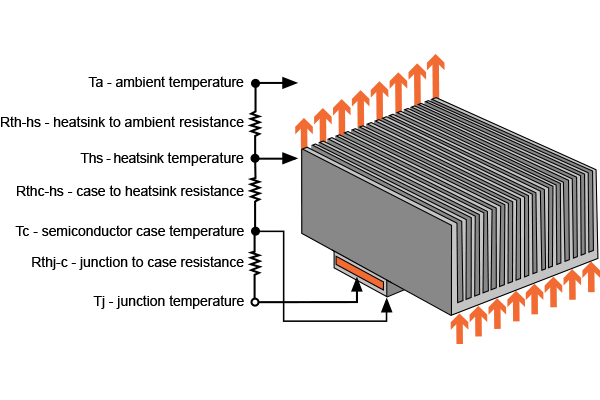# Heat Sink Calculator

## This calculator helps determine the maximum temperature a device can withstand

°C
°C
°C/Watt
°C/Watt
°C/Watt

Watts
°C

### How does the Heat Sink Calculator Work?

This tool is designed to calculate junction temperature of an electronic device (typically power devices) given four parameters: the maximum ambient temperature, the device's junction-to-package thermal resistance, the thermal resistance of the heat sink, and the power applied. It can also calculate the maximum power that the device can handle given its junction temperature, the maximum ambient temperature, the junction-to-package thermal resistance, and the heat sink's thermal resistance.#### Equations

$$T_{J}= P(R_{case}+R_{1}+R_{2})+T_{a}$$

Where:

$$T_{J}$$ = junction temperature

$$P$$ = power dissipated

$$R_{case}$$ = thermal resistance of device junction to case

$$R_{1}$$ = thermal resistance of device junction to air (if no heat sink) or thermal resistance of heat sink

$$R_{2}$$ = thermal resistance of device junction to air

### Applications of a Heat Sink Calculator

The highest operating temperature that a semiconductor in an electronic device can withstand is called its junction temperature. This temperature is commonly higher than the temperature of the device's exterior and its case. The heat transferred from the junction to case, multiplied by the junction-case thermal resistance, is equal to the difference between the junction temperature and the outside, plus, case temperature.

The maximum junction temperature of a device is always found on its datasheet. It can be very useful when there is a need to compute for the required case-ambient thermal resistance, given the amount of dissipated power. The value of the maximum junction temperature is then used for choosing the right heat sink.

Microprocessors often measure the temperature of its core through a sensor. When the core reaches its maximum junction temperature, a cooling mechanism is triggered. Also, if the temperature goes beyond the maximum junction temperature, an alarm will be triggered that warns the computer operator to discontinue the process that is causing the overheating of the processor's core.

### Notes

#### Typical values of Thermal Resistance for Various Electronics Packages

 Package Junction to Case (°C/Watt) Junction to Air (°C/Watt) TO-3 5 60 TO-39 12 140 TO-220 3 62.5 TO-220FB 3 50 TO-223 30.6 53 TO-252 5 92 TO-263 23.5 50 D2PAK 4 35

#### Thermal Resistance for PCB Copper

 Heat Sink Thermal resistance (°C/Watt) 1 sq inch of 1 ounce PCB copper 43 .5 sq inch of 1 ounce PCB copper 50 .3 sq inch of 1 ounce PCB copper 56 Aavid Thermalloy, SMT heat sink:  PN:573400D00010 14